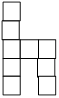### Sample Problem

The figure ‘h’ is made up of 9 identical squares (assume that the figure is evenly-lined) . The total length of the lines needed to draw the figure is 40 cm. The area of the figure is cm 2#### Solution

First we should find out how many sides there are in the figure. There are 20 sides of the small squares, and the total length of all the sides is cm, so each side has length 40 ÷ 20 = 2 cm. So the area of the figure is 2 × 2 × 9 = 36 cm 2